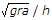# Mechanical Engineering - Engineering Mechanics

### Exercise :: Engineering Mechanics - Section 2

1.

The point, through which the whole weight of the body acts, irrespective of its position, is known as

 A. moment of inertia B. centre of gravity C. centre of percussion D. centre of mass

Explanation:

No answer description available for this question. Let us discuss.

2.

The loss of kinetic energy during elastic impact is zero.

 A. Agree B. Disagree

Explanation:

No answer description available for this question. Let us discuss.

3.

The overturning of a vehicle on a level circular path can be avoided if the velocity of vehicle is __________.

 A. less than B. greater than

Explanation:

No answer description available for this question. Let us discuss.

4.

Which of the following is a scalar quantity?

 A. Force B. Speed C. Velocity D. Acceleration

Explanation:

No answer description available for this question. Let us discuss.

5.

The rate of change of momentum is directly proportional to the impressed force, and takes place in the same direction in which the force acts. This statement is known as

 A. Newton's first law of motion B. Newton's second law of motion C. Newton's third law of motion D. none of these